Prev: 5.3 Coupling with Lithography Up: 5.3 Coupling with Lithography Next: 5.3.2 Contact Hole Printing

## 5.3.1 Lithography Modeling

Optical lithography is a complex process determined by many chemical and physical effects. As indicated in Fig. 5.5, a rigorous model for the simulation of photo-lithographic exposure has to include many cross-related quantities. The concentration of the photo-active compound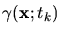changes with the incident light intensity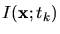and determines the optical properties of the resist, such as the permittivity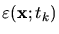and the refractive index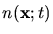. Hence, the electro-magnetic phasor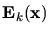has to be calculated inside an inhomogeneous medium. The absorbed light intensity retro-acts on the concentration of the photo-active compound, which requires an iterative solution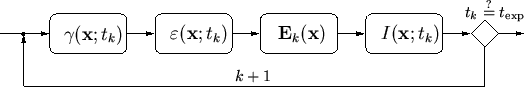The crucial point throughout lithography simulation is the solution of the Maxwell equations. Several methods for the numerical solution of the Maxwell equations have been proposed, ranging from simple vertical scalar models to rigorous approaches based on FEM discretizations of the Maxwell equations. We have used a three-dimensional extension of the differential method , which will be recapitulated in the following.

As can be seen from Fig. 5.5, the absorbed light intensity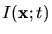inside the optically nonlinear resist has to be determined. The exposure state of the photo-resist is described by the concentration of the photo-active compound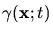. Dill's ABC''-model  is used for the correlation between the exposure intensityand the bleaching of the resist, which determines the change in the resist's refractive index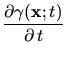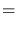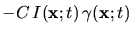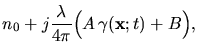(5.4)

where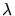is the wavelength used for the exposure. This relation requires the knowledge about the intensity distribution which has to be calculated from the solution of the Maxwell equations. Assuming a time-harmonic field distribution within a time-step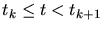, the EM field obeys the Maxwell equations in the form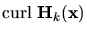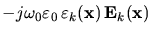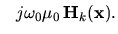(5.5)

Due to the spatially periodic nature of the incident light and the assumption of a laterally periodic simulation domain the EM field inside the simulation domain can be expressed by a Fourier expansion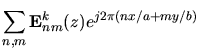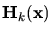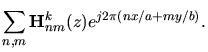(5.6)

The permittivity itself is related to the refractive index by Maxwell's formula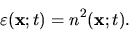(5.7)

Additionally, the inhomogeneous permittivity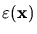and its reciprocal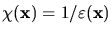can be expanded in Fourier series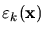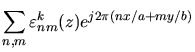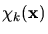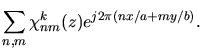(5.8)

Insertion of (5.6) and (5.8) into (5.5) transforms the partial differential equations (PDE) into an infinite-dimensional set of coupled ordinary differential equations (ODE) for the Fourier coefficients of the lateral field components.

For the numerical solution of the equation system, the application of appropriate boundary conditions is necessary. Above the simulation domain we have to consider incident and reflected waves, whereas below only outgoing waves occur. The incident light is known from the aerial image simulation, whereas the unknown reflected and outgoing fields are eliminated by applying radiation boundary conditions. The resulting boundary value problem is solved by a numerically efficient implementation of the shooting method  which supplies the EM field coefficients.

The EM field coefficients are transformed back to the spatial domain and the solution of the EM field intensity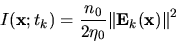(5.9)

for the time-step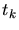is obtained. In case of strongly bleaching resists this process has to be repeated until the total exposure dose is reached.

Because the bleaching rateis almost negligible when compared with the frequency of the EM field, the refractive indexvaries only slowly with respect to the field propagation and thus a quasi-static approximation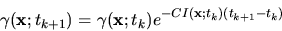(5.10)

can be applied to (5.4). The inhomogeneous PAC concentration can be derived from this expression. As last step, we use Kim's R''-model  to relate the PAC distribution to a spatially inhomogeneous development rate. The numerical values of the development rate are given on a tensor product grid which is used for the representation of the optical properties of the simulated structure. If the spacing of the grid is the same as the cell size used for the topography simulation, the rates can be transfered directly to the cellular structure, otherwise the rates have to be interpolated. In the following section this simulation approach will be applied to the printing of a contact hole over different substrates.

W. Pyka: Feature Scale Modeling for Etching and Deposition Processes in Semiconductor Manufacturing